# Rao-Blackwell-Kolmogorov theorem

(diff) ← Older revision | Latest revision (diff) | Newer revision → (diff)

A proposition from the theory of statistical estimation on which a method for the improvement of unbiased statistical estimators is based.

Letbe a random variable with values in a sample space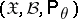,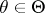, such that the family of probability distributions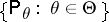has a sufficient statistic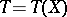, and letbe a vector statistic with finite matrix of second moments. Then the mean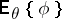ofexists and, moreover, the conditional mean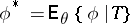is an unbiased estimator for, that is,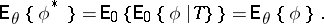The Rao–Blackwell–Kolmogorov theorem states that under these conditions the quadratic risk of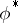does not exceed the quadratic risk of, uniformly in, i.e. for any vectorof the same dimension as, the inequalityholds for any. In particular, ifis a one-dimensional statistic, then for anythe variance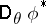ofdoes not exceed the variance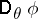of.

In the most general situation the Rao–Blackwell–Kolmogorov theorem states that averaging over a sufficient statistic does not lead to an increase of the risk with respect to any convex loss function. This implies that good statistical estimators should be looked for only in terms of sufficient statistics, that is, in the class of functions of sufficient statistics.

In case the family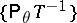is complete, that is, when the function ofthat is almost-everywhere equal to zero is the only unbiased estimator based onfor zero, the unbiased estimator with uniformly minimal risk provided by the Rao–Blackwell–Kolmogorov theorem is unique. Thus, the Rao–Blackwell–Kolmogorov theorem gives a recipe for constructing best unbiased estimators: one has to take some unbiased estimator and then average it over a sufficient statistic. That is how the best unbiased estimator for the distribution function of the normal law is constructed in the following example, which is due to A.N. Kolmogorov.

Example. Given a realization of a random vector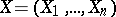whose components,,, are independent random variables subject to the same normal law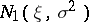, it is required to estimate the distribution function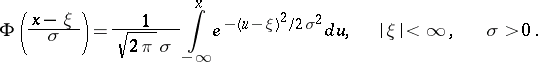The parametersandare supposed to be unknown. Since the family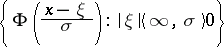of normal laws has a complete sufficient statistic, where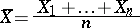andthe Rao–Blackwell–Kolmogorov theorem can be used for the construction of the best unbiased estimator for the distribution function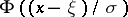. As an initial statisticone may use, e.g., the empirical distribution function constructed from an arbitrary componentof: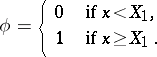This is a trivial unbiased estimator for, since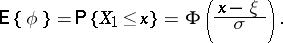Averaging ofover the sufficient statisticgives the estimator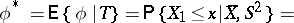(1)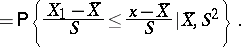Since the statistic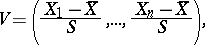which is complementary to, has a uniform distribution on the-dimensional sphere of radiusand, therefore, depends neither on the unknown parametersand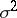nor on, the same is true for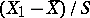and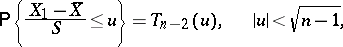(2)

where(3)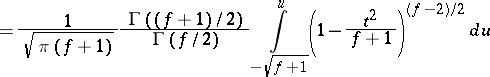is the Thompson distribution withdegrees of freedom. Thus, (1)–(3) imply that the best unbiased estimator forobtained fromindependent observationsis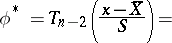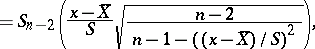where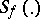is the Student distribution withdegrees of freedom.